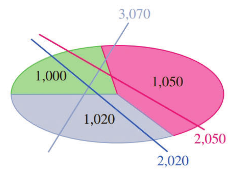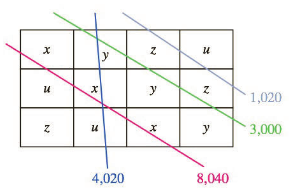Chapter 4.3, Problem 42EFinite Mathematics and Applied Cal...

7th Edition
Stefan Waner + 1 other
ISBN: 9781337274203

Solutions

Chapter
SectionFinite Mathematics and Applied Cal...

7th Edition
Stefan Waner + 1 other
ISBN: 9781337274203
Textbook Problem

CAT Scans CAT (computerized axial tomographic) scans are used to map the exact location of interior features of the human body. CAT scan technology is based on the following principles: (I) Different components of the human body (water; gray matter; bone, etc.) absorb X-rays to different extents; and (2) to measure the X-ray absorption by a specific region of, say, the brain, it suffices to pass a number of line-shaped pencil beams of X-rays through the brain at different angles and measure the total absorption for each beam, which is the sum of the absorptions of the regions through which it passes. The accompanying diagram illustrates a simple example. (The number in each region shows its absorption, and the number on each X-ray beam shows the total absorption for that beam.)27In Exercises 39-44, use the table and the given X-ray absorption diagrams to identity the composition of each of the regions marked by a letter. Type Air Water Gray Matter Tumor Blood Bone Absorption 0 1,000 1,020 1,030 1,050 2,000To determine

To calculate: The composition of each of the regions marked by a letters x, y, z and z with the help of provided diagram and table.

Explanation

Given Information:

The provided table is shown below:

 Type Air Water Grey matter Tumor Blood Bone Absorption 0 1000 1020 1030 1050 2000

The provided X-ray diagram is shown below:

Formula Used:

Elementary row operations

Type 1: Replacing the row Ri by aRi, where a is a nonzero number.

Type 2: Replacing the row Ri by aRi±bRj, where a is a nonzero number.

Gauss Jordan reduction method:

Step 1: First clear the fractions or decimals if any, using operations of type 1.

Step 2: Select the first nonzero element of the first row as pivot.

Step 3: Use the pivot to clear its column using operations of type 2.

Step 4: Select the first nonzero element in the second row a pivot and clear its column.

Step 5: Turn all the selected pivot elements into a 1 using operations of type 1.

Calculation:

Consider the provide table is,

 Type Air Water Grey matter Tumor Blood Bone Absorption 0 1000 1020 1030 1050 2000

Consider the provided diagram is,

To determine the values of each compositions equations can be formed by adding the values along each beams.

Add the values along each beam.

x+y+u=4020

And

3x+2u=8040

Also,

3y+2z=3000

And

2z+u=1020

The augmented matrix for the given system of equations:



Apply Gauss Jordan reduction method to get the solution of the given system of equation.

Pivot the first nonzero element of the first row and clear its column.

Perform the operations R2R23R1.



Pivot the first nonzero element of the second row and clear its column.

Perform the operations R13R1+R2, R3R3+R2.



Next pivot the first nonzero element of the third row and clear its column.

Perform the operations R4R4R3,



In the next step,

Perform the operation R412R4

Still sussing out bartleby?

Check out a sample textbook solution.

See a sample solution

The Solution to Your Study Problems

Bartleby provides explanations to thousands of textbook problems written by our experts, many with advanced degrees!

Get Started